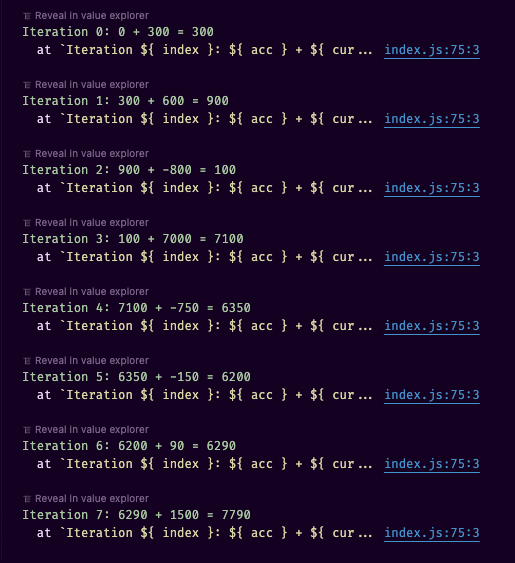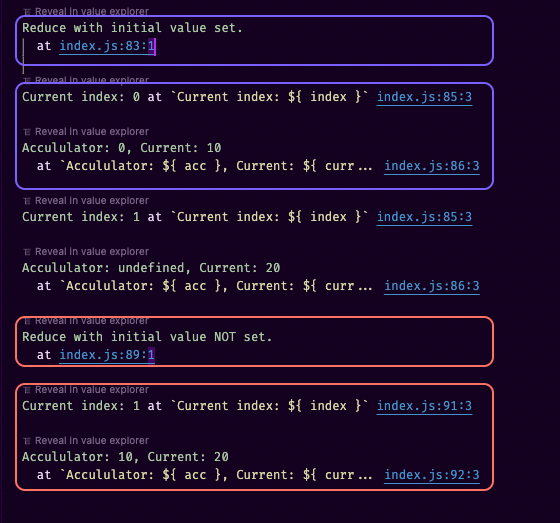# const - JS | lectureWorking With Arrays

The reduce Method

In this lecture, we'll talk about the third data transformation method; the reduce method. And as you will remember, we use the reduce method to essentially boil down all the elements in an array to one single value. And we talked about the example of adding up all the numbers in one array.

Let's try that now with our `movements` array.

script.js
``const movements = [300, 600, -800, 7000, -750, -150, 90, 1500];``

By adding up all the numbers in the array, both the deposits and the withdrawals, we will end up will a global balance. This means we can have something like this:

script.js
``const balance = movements.reduce();``

Remember that what's returned in the `balance` variable is simply one value, not an entire array.

Just like the map and filter methods, the reduce method has a callback function that is called for each element in the array. But this one is a little bit different. In the map and filter methods, the first parameter is always the current element in the array, the second one is the index of the current element, and the third one is the array itself. But in the reduce method, the first parameter is something called the accumulator. Then the remaining parameters are the current element in the array, the index of the current element, and the array itself.

script.js
``````/**
* In most documentation, the accumulator is abbreviated as `acc`
*/
const balance = movements.reduce(function (
accumulator,
currentElement,
index,
movements
) {
// do something...
});``````

The accumulator is like a snowball that keeps on accumulating the value that we ultimately want to return. Considering the case of adding up all the numbers in the array, the accumulator will be the sum of all the numbers.

We've learned that the reduce method accepts a callback that executed for each element in the array, and that this callback has an accumulation. But now what next? Well, since the accumulator is the value that we will keep adding to, what we need to do is to add the current element to the accumulator.

script.js
``````const balance = movements.reduce(function (acc, currentElement) {
return acc + currentElement;
});``````

The `return` statement is important because in each loop iteration, the accumulator is updated with the new value.

Next,the reduce method actually has a second parameter, which is the initial value of the accumulator in the first loop iteration. In our case, we want to start counting from 0.

script.js
``````const balance = movements.reduce(function (acc, currentElement) {
return acc + currentElement;
}, 0);``````

Let's now check our balance.

script.js
``console.log(balance); // => 7790``

If your array is the same as mine, you should have a balance of 7790. To make this even more clear, let's log the accumulator in each loop iteration.

script.js
``````const balance = movements.reduce(function (acc, currentElement, index) {
console.log(
`Iteration \${index}: \${acc} + \${currentElement} = \${acc + currentElement}`
);
return acc + currentElement;
}, 0);

console.log(balance);``````We can see that at iteration 0, the accumulator is 0 because that's the value that we specified as the initial value. Then at iteration 1, the accumulator is already at 300. And that's because, that is the initial value plus the current element in the previous iteration (300). Still in iteration 1, we add the current element again, which is 600. And therefore, in the next iteration, that is the value of the accumulator.

Hope you can now see what I meant by the snowball effect. All these values adding up to a single value. And in the end, that value is essentially the accumulator. I hope the above log is clear enough to understand the reduce method.

Let's one more time do the same thing with the for-of loop.

script.js
``````let balance = 0; // This represents the accumulator with the initial value of 0
for (const movement of movements) {
console.log(`\${balance} + \${movement} = \${balance + movement}`);
balance += movement;
}

console.log(balance); // => 7790``````

You can see this common pattern that we always need an external variable whenever we want to use a for loop. And that's totally fine if you only need one loop, but it starts to become really cumbersome and unpractical when we use many loops for doing many operations. So these methods that we've been studying, they completely avoid this problem of creating an external variable. And they simply return the value right away.

To finish this lecture, let me show you one final example because we can do more than just adding up all the numbers in an array. This time what I want us to do is to get the maximum value of the `movements` array. So in this case, the result we're looking for is 7000.

Remember that reduce is for boiling down the array into just one single value, but that value can be whatever we want. It doesn't have to be a sum. It could be a multiplication, or even something completely different, like a string or an object. But, here we will keep working with numbers. And right now we want the maximum number in the array.

If we had to do this manually while talking to ourselves, we would have this:

``````-- Starting with 300, is it the maximum value at this point? 🤔
- Well, we don't know yet because we just started, let me just save it in my brain 🧠 as the current maximum value.
-- Then 600, is it greater than the current maximum value which was 300? 🤔
- Yes, it is. Let me save that as the new maximum value.
-- Then -800, is it greater than the current maximum value which was 600? 🤔
- No, it isn't. Let me move on to the next element. ⏭
-- Then 7000, is it greater than the current maximum value which was 600? 🤔
- Yes, it is. Let me save that as the new maximum value.
-- Then -750, is it greater than the current maximum value which was 7000? 🤔
- No, it isn't. Let me move on to the next element. ⏭
-- Then -150, is it greater than the current maximum value which was 7000? 🤔
- No, it isn't. Let me move on to the next element. ⏭
-- Then 90, is it greater than the current maximum value which was 7000? 🤔
- No, it isn't. Let me move on to the next element. ⏭
-- Then 1500, is it greater than the current maximum value which was 7000? 🤔
- No, it isn't. Let me move on to the next element. ⏭

That was the last element. So the maximum value is 7000. 😁``````

Now let's translate this to code using the reduce method.

script.js
``````const maximumValue = movements.reduce((acc, curr) => acc > curr ? acc : curr, 0);

// OR

// curr is the current movement
const maximumValue = movements.reduce((acc, curr) => {
if (acc > curr) {
return acc;
} else {
return curr;
}
}, 0);
``````

Two things to note here. First, you should always ask yourself what is the purpose of the accumulator. Above when we were adding up all the numbers, the purpose of the accumulator was to keep track of the current sum. But now, we want to keep track of the current maximum value.

Secondly, you've noticed that my initial value is 0. The ideal solution is always to start with the first element in the array when trying to find a maximum or minimum. And, there are two ways to do that.

script.js
``````// Manually setting the first element as the initial value
const maximumValue = movements.reduce((acc, curr) => acc > curr ? acc : curr, movements);

// OR

// Initial value not set
const maximumValue = movements.reduce((acc, curr) => acc > curr ? acc : curr);``````

By manually setting the first element as the initial value, or when you just set an initial value, the current element is the first element in the array and the current index starts at 0. If you don't set an initial value, the accumulator is the first element in the array, the current element is the second element in the array, and the current index starts at 1.

Let's have this simple example to prove what I'm saying.

script.js
``````const simpleArray = [10, 20]
console.log('Reduce with initial value set.');
simpleArray.reduce((acc, curr, index) => {
console.log(`Current index: \${index}`);
console.log(`Accululator: \${acc}, Current: \${curr}`);
}, 0);

console.log('Reduce with initial value NOT set.');
simpleArray.reduce((acc, curr, index) => {
console.log(`Current index: \${index}`);
console.log(`Accululator: \${acc}, Current: \${curr}`);
});``````To finish this lecture, you have to know that we can do many things with this reduce method. It is by far the most powerful array method there is. And because of that, it can also be the hardest one to use. So we always need to consider exactly what we want the accumulator and the current value to be and how they should interact. But I hope that with the examples provided, I could demonstrate the thought process a little bit, and throughout the course, there will be some more exercises of the reduce method so that you can also learn better and better how to use it yourself one day.